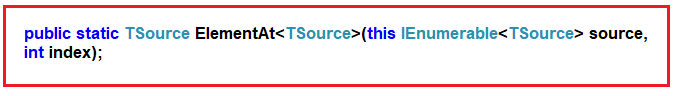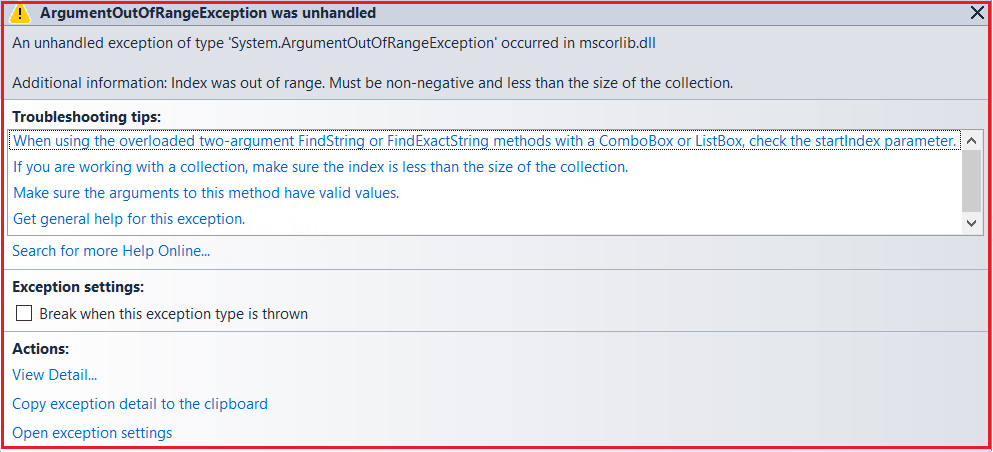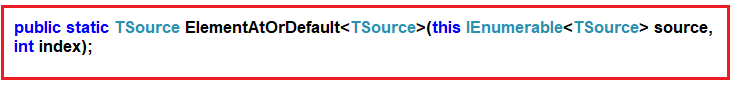# ElementAt and ElementAtOrDefault in Linq

## ElementAt and ElementAtOrDefault in Linq

In this article, I am going to discuss the ElementAt and ElementAtOrDefault operator in Linq with some examples. Please read our previous article before proceeding to this article, where we discussed the basics of Element Operators in Linq.

##### ElementAt Operator in Linq:

The ElementAt operator is used to return an element from a specific index. If the data source is empty or if the provided index value is out of range, then we will get ArgumentOutOfRangeException. Let us see the signatures of this method as shown below.As you can see, this method takes one parameter i.e. the index position. Then it will return the element present in that index position of the data source. There is no overloaded version available for this method.

##### Example1: Return the element present in index position 1.

Please have a look at the following program. Here we have created one data source which contains integer numbers. Then we fetch the element present in index position 1 by using the ElementAt method and to that method, we pass the value 1.

```using System.Linq;
using System;
using System.Collections.Generic;
namespace LINQJoin
{
class Program
{
static void Main(string[] args)
{
List<int> numbers =new List<int>() { 1, 2, 3, 4, 5, 6, 7, 8, 9, 10 };
int MethodSyntax = numbers.ElementAt(1);
Console.WriteLine(MethodSyntax);

}
}
}
```

Run the application and it should print 2 in the output window as in index position 1 the value 2 is there.

##### Example2: Index Out of Range

As our data source contains 10 elements so the index is going to start from 0 to 9. Now let see what happen I try to fetch the element from index position 10 or try to pass a negative index value as shown below.

```using System.Linq;
using System;
using System.Collections.Generic;
namespace LINQJoin
{
class Program
{
static void Main(string[] args)
{
List<int> numbers =new List<int>() { 1, 2, 3, 4, 5, 6, 7, 8, 9, 10 };
//int MethodSyntax = numbers.ElementAt(-1);
int MethodSyntax = numbers.ElementAt(10);
Console.WriteLine(MethodSyntax);

}
}
}
```

Now if you run the application, then you will get the following exception.##### Example3: Empty Data source

Let see what happens if we apply the ElementAt operator on an empty data source as shown in the following example.

```using System.Linq;
using System;
using System.Collections.Generic;
namespace LINQJoin
{
class Program
{
static void Main(string[] args)
{
List<int> numbers =new List<int>() { };
int MethodSyntax = numbers.ElementAt(1);
Console.WriteLine(MethodSyntax);

}
}
}
```

Again you will get the same exception as the previous example.

Note: If the data source is empty or if you specify a negative value for the index position or if you specify the index position which is out of range then you will get a runtime exception.

If you don’t want that exception then you need to use the ElementAtOrDefault method.

##### ElementAtOrDefault Method in Linq:

The ElementAtOrDefault method does the same thing as the ElementAt method except that this method does not throw an exception when the data source is empty or when the supplied index value is out of range. In such cases, it will return the default value based on the data type of the element the data source contain. Please have a look at the definition of this method as shown in the below image.Like the ElementAt method, this method also does not have an overloaded version. Let us understand this method with some examples.

##### Example4: Fetch the element from index position 1.
```using System.Linq;
using System;
using System.Collections.Generic;
namespace LINQJoin
{
class Program
{
static void Main(string[] args)
{
List<int> numbers =new List<int>() { 1, 2, 3, 4, 5, 6, 7, 8, 9, 10 };
int MethodSyntax = numbers.ElementAtOrDefault(1);
Console.WriteLine(MethodSyntax);

}
}
}
```

Output: 2

##### Example5: Fetch the element from index position 10.
```using System.Linq;
using System;
using System.Collections.Generic;
namespace LINQJoin
{
class Program
{
static void Main(string[] args)
{
List<int> numbers =new List<int>() { 1, 2, 3, 4, 5, 6, 7, 8, 9, 10 };
int MethodSyntax = numbers.ElementAtOrDefault(10);
Console.WriteLine(MethodSyntax);

}
}
}
```

Output: 0

Here it will print the value 0. This is because the data source contains integers. And the default for integer is 0.

##### Example6: Using Query Syntax

There is no such operator call ElementAt Or ElementAtOrDefault is available to write the query syntax, If you want then you can combine both the method syntax and query syntax to write the code as shown below.

```using System.Linq;
using System;
using System.Collections.Generic;
namespace LINQJoin
{
class Program
{
static void Main(string[] args)
{
List<int> numbers = new List<int>() { 1, 2, 3, 4, 5, 6, 7, 8, 9, 10 };

int QuerySyntax1 = (from num in numbers
select num).ElementAt(1);

int QuerySyntax2 = (from num in numbers
select num).ElementAtOrDefault(1);

Console.WriteLine(QuerySyntax1);
Console.WriteLine(QuerySyntax2);